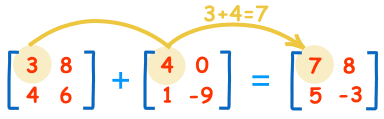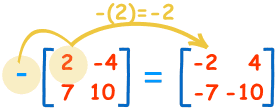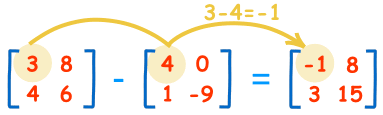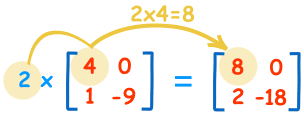# Matrices

A Matrix is an array of numbers:A Matrix
(This one has 2 Rows and 3 Columns)

We talk about one matrix, or several matrices.

There are many things we can do with them ...

To add two matrices: add the numbers in the matching positions:These are the calculations:
 3+4=7 8+0=8 4+1=5 6−9=−3

The two matrices must be the same size, i.e. the rows must match in size, and the columns must match in size.

Example: a matrix with 3 rows and 5 columns can be added to another matrix of 3 rows and 5 columns.

But it could not be added to a matrix with 3 rows and 4 columns (the columns don't match in size)

## Negative

The negative of a matrix is also simple:These are the calculations:
 −(2)=−2 −(−4)=+4 −(7)=−7 −(10)=−10

## Subtracting

To subtract two matrices: subtract the numbers in the matching positions:These are the calculations:
 3−4=−1 8−0=8 4−1=3 6−(−9)=15

Note: subtracting is actually defined as the addition of a negative matrix: A + (−B)

## Multiply by a Constant

We can multiply a matrix by a constant (the value 2 in this case):These are the calculations:
 2×4=8 2×0=0 2×1=2 2×−9=−18

We call the constant a scalar, so officially this is called "scalar multiplication".

## Multiplying by Another Matrix

To multiply two matrices together is a bit more difficult ... read Multiplying Matrices to learn how.

## Dividing

And what about division? Well we don't actually divide matrices, we do it this way:

A/B = A × (1/B) = A × B-1

where B-1 means the "inverse" of B.

So we don't divide, instead we multiply by an inverse.

And there are special ways to find the Inverse, learn more at Inverse of a Matrix.

## Transposing

To "transpose" a matrix, swap the rows and columns.

We put a "T" in the top right-hand corner to mean transpose:## Notation

A matrix is usually shown by a capital letter (such as A, or B)

Each entry (or "element") is shown by a lower case letter with a "subscript" of row,column:### Rows and Columns

So which is the row and which is the column?

• Rows go left-right
• Columns go up-down

To remember that rows come before columns use the word "arc":

ar,c

### Example:

 B =Here are some sample entries:

b1,1 = 6 (the entry at row 1, column 1 is 6)

b1,3 = 24 (the entry at row 1, column 3 is 24)

b2,3 = 8 (the entry at row 2, column 3 is 8)